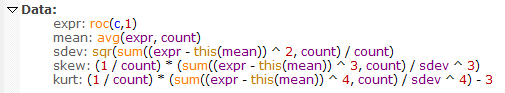Navigation: Realtest Script Language > Syntax Element Details > StdDev

Category

Multi-Bar Functions

Description

Statistical measure of the variance of a distribution of values

Syntax

StdDev(expr, count)

Parameters

expr - data series formula

count - lookback period

Notes

StdDev is calculated in the same way that Excel calculates the STDEV.P function, which is as if the set of count values is the entire population.

The specific formula used is shown below, in the "sdev" item:This also illustrates how these statistical functions could be calculated in the Data Section of a script, though since they're provided built-in, there's no reason to do so.